Question

# 5. Consider the complex number z 3i Find the polar form Find z4. Put in non-polar form a. b. 5. Consider the complex number z 3i Find the polar form Find z4. Put in non-polar form a. b.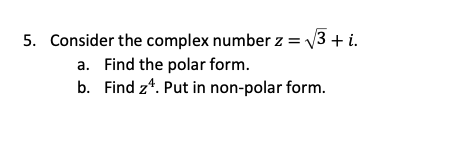5. Consider the complex number z 3i Find the polar form Find z4. Put in non-polar form a. b.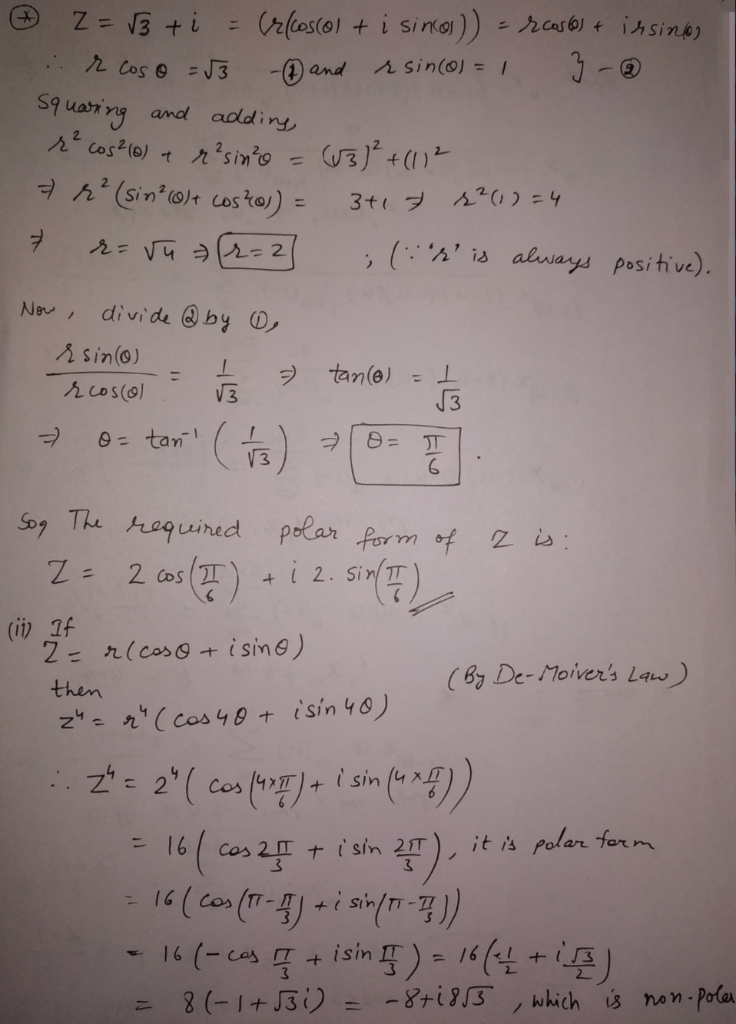#### Earn Coins

Coins can be redeemed for fabulous gifts.

Similar Homework Help Questions
• ### 14 For the complex number z=-3+3i do the following a) Convert to polar form, Polar 2-...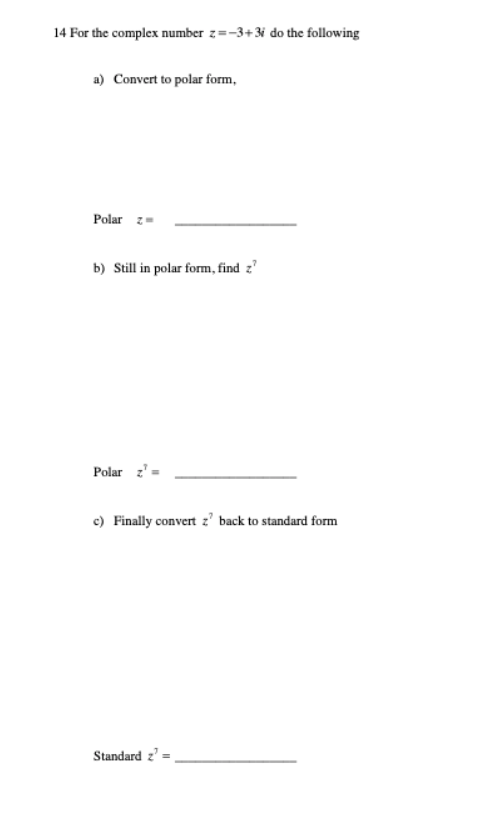14 For the complex number z=-3+3i do the following a) Convert to polar form, Polar 2- b) Still in polar form, find z? Polar c) Finally convert z' back to standard form Standard 2' =

• ### 3i)16 in polar form: z r(cos 0isin 0) where (1 Write the complex number z and...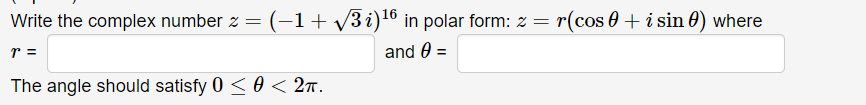3i)16 in polar form: z r(cos 0isin 0) where (1 Write the complex number z and e= The angle should satisfy 0 0 < 2«.

• ### Use the polar form of the complex number 5 i to find a value in Cartesian...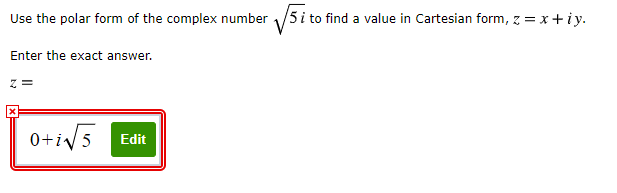Use the polar form of the complex number 5 i to find a value in Cartesian form, z = x+iy. Enter the exact answer. Z= 0+iv 5 Edit

• ### For the complex number given as: z = a + bi / c+di where i =...

For the complex number given as: z = a + bi / c+di where i = √−1 is the imaginary unit. The parameters are defined as a = √2, b = 0, c = 0.5 and d = −0.5. (a) Find the real and the imaginary parts of z, and then draw the Argand dia- gram. (Hint: Use the conjugate of the denominator.) 2.5 (b) Based on the Argand diagram, find the distance r of the complex number z from...

• ### complex numbers son a) Express Z as a complex number in rectangular form. Z = (5...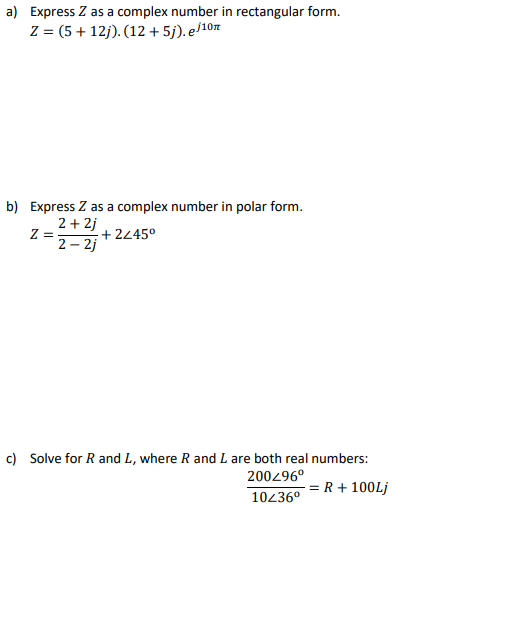complex numbers son a) Express Z as a complex number in rectangular form. Z = (5 + 12j).(12 + 5j). e 10 b) Express Z as a complex number in polar form. 2+2+2245° 2=2-2j c) Solve for R and L, where R and L are both real numbers: 200296 + 100Li 102360R

• ### 3. Find the polar form of each given complex number and sketch its position in the...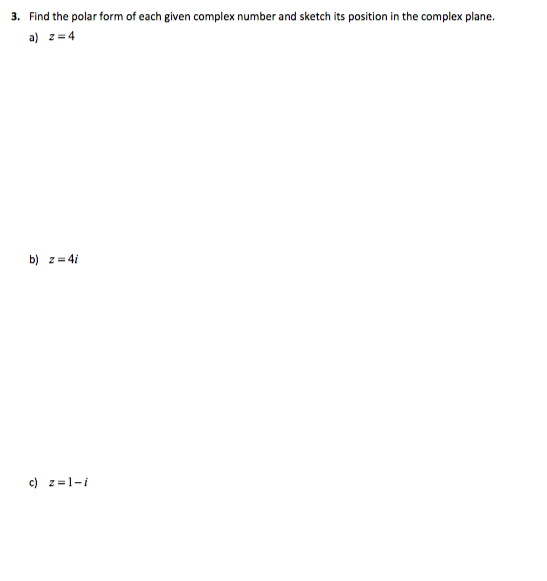3. Find the polar form of each given complex number and sketch its position in the complex plane. a) 2-4 b) z 4i c) 2-1-i

• ### Express the complex number 10 - 24i in polar form, z = rei, where - <O<T....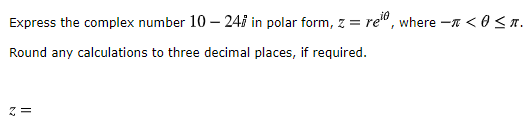Express the complex number 10 - 24i in polar form, z = rei, where - <O<T. Round any calculations to three decimal places, if required.

• ### Plot the complex number. Then write the complex number in polar form. Express the argument as...

Plot the complex number. Then write the complex number in polar form. Express the argument as an angle between 0 degrees and 360 degrees . 1-4i z=___(cos__+i sin__)

• ### Express the complex number z= in polar form เรเเ uLliuus. ru eaa. yusuun, suuw au wurx...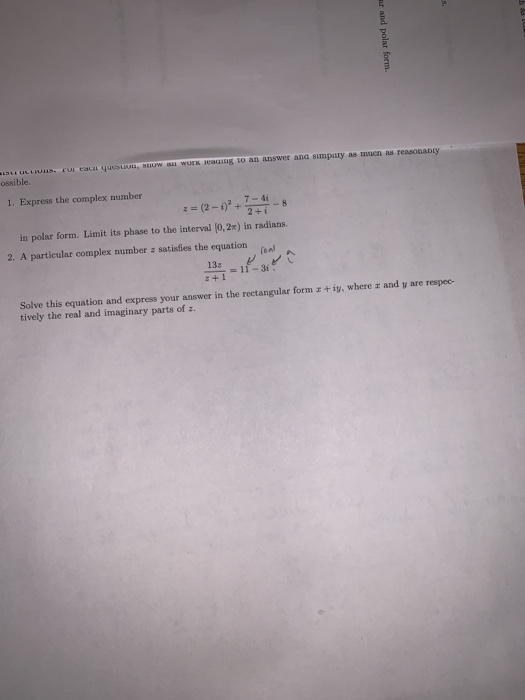Express the complex number z= in polar form เรเเ uLliuus. ru eaa. yusuun, suuw au wurx eauug to an answer and simpiny as mucn as reasonably 1. Express the complex number 7-4i in polar form. Limit its phase to the interval [0, 2m) in radians. 2. A particular complex number z satisfles the eqio z+ 1 Solve this equation and express your answer in the rectangular form a +iy, where z and y are respec tively the real and imaginary...

• ### Plot the complex number on the complex plane and write it in polar form and in...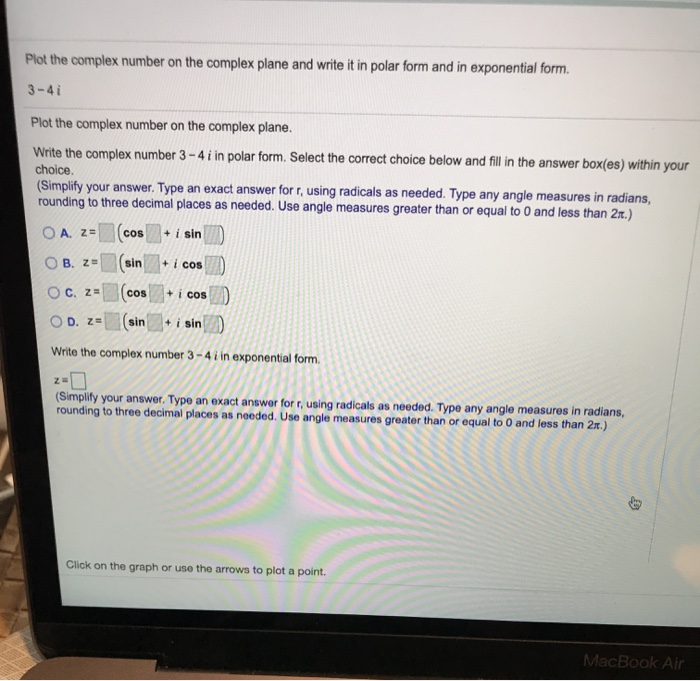Plot the complex number on the complex plane and write it in polar form and in exponential form. 3-41 Plot the complex number on the complex plane. Write the complex number 3 - 4 i in polar form. Select the correct choice below and fill in the answer box(es) within your choice. (Simplify your answer. Type an exact answer for r, using radicals as needed. Type any angle measures in radians, rounding to three decimal places as needed. Use angle...